Menu Outline Exercise Questions Appendices Lectures Student Projects Books Sections Introduction Atoms Molecules Crystal Structure Crystal Diffraction Crystal Binding Photons Phonons Electrons Band Model Crystal Physics Semiconductors

PHY.K02UF Molecular and Solid State Physics

## Two dimensional periodic functions

A periodic function consists of a primitive unit cell that is repeated on a Bravais lattice. There are five Bravais lattices in two dimensions.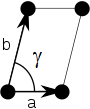ObliqueRectangularCentered rectangularSquareHexagonal

A Bravais lattice can be specified by giving two primitive lattice vectors, $\vec{a}_1$ and $\vec{a}_2$. The lattice parameters (or lattice constants) in two dimensions are the lengths of the primitive lattice vectors $a = |\vec{a}_1|$, $b=|\vec{a}_2|$, and the angle $\gamma$ between the primitive lattice vectors, $\cos\gamma = \vec{a}_1\cdot\vec{a}_2/(ab)$. For convenience $\vec{a}_1$ can be chosen to point in the $x-$direction,

$$\vec{a}_1 = a\,\hat{x},\qquad\vec{a}_2 = b\cos\gamma\,\hat{x} + b\sin\gamma\,\hat{y}.$$

The primitive lattice vectors in reciprocal space can then be calculated using the conditions,

$$\vec{a}_i\cdot\vec{b}_j=2\pi\delta_{ij}.$$

The primitive lattice vectors in reciprocal space are,

$$\vec{b}_1 = \frac{2\pi}{a}\,\hat{k_x}-\frac{2\pi\cos\gamma}{a\sin\gamma}\,\hat{k_y},\qquad\vec{b}_2 = \frac{2\pi}{b\sin\gamma}\,\hat{k_y}.$$

A Fourier series can be used to describe a periodic function in two dimensions. The expression for a Fourier series is,

$$f(\vec{r})=\sum \limits_{\vec{G}} f_{\vec{G}}\exp \left(i\vec{G}\cdot\vec{r}\right).$$

The reciprocal lattice vectors are $\vec{G}_{\nu_1,\nu_2}=\nu_1\vec{b}_1+\nu_2\vec{b}_2$, where $\nu_1$ and $\nu_2$ are integers. The Fourier coefficients can be specified with the same integers $f_{\nu_1,\nu_2}=f_{\vec{G}_{\nu_1,\nu_2}}$

A two-dimensional periodic function is plotted below. The form can be used to adjust the ratio $b/a$ and the angle $\gamma$. It is possible to specify four Fourier coefficients $f_{\nu_1,\nu_2}$. The Fourier coefficients are set such that $f_{\vec{G}} = f^*_{\vec{G}}$ so that the periodic function is real. All other Fourier coefficients are set to zero.

 $b/a=$ $\gamma=$ °

$\vec{a}_1=1\,\hat{x}$   $\vec{a}_2=$ $\hat{x} + ($ $) \hat{y}$

$\vec{b}_1=$ $\hat{k}_x + ($ $)\hat{k}_y$   $\vec{b}_2=$ $\hat{k}_y$

 $f_{00}=$ $f_{01}=f^*_{0-1}=$ $+i$ () $f_{10}=f^*_{-10}=$ $+i$ () $f_{11}=f^*_{-1-1}=$ $+i$ () $f_{1-1}=f^*_{-11}=$ $+i$ ()

Determining the Fourier coefficients

If the periodic function $f(\vec{r})$ is known, the Fourier coefficients $f_{\vec{G}}$ can be determined by multiplying both sides of a Fourier series by $\exp (-i\vec{G}'\cdot \vec{r})$ and integrating over a primitive unit cell.

$$\int\limits_{\text{unit cell}}f(\vec{r})\exp (-i\vec{G}'\cdot \vec{r})d\vec{r} = \sum\limits_{\vec{G}}\int\limits_{\text{unit cell}}f_{\vec{G}}\exp (-i\vec{G}'\cdot \vec{r})\exp (i\vec{G}\cdot \vec{r})d\vec{r}$$

On the right-hand side, only the term where $\vec{G} = \vec{G}'$ contributes and the integral evaluates to $f_{\vec{G}}$ times the volume $V_{\text{uc}}$ of the primitive unit cell.

$$f_{\vec{G}} = \frac{1}{V_{\text{uc}}}\int\limits_{\text{unit cell}}f(\vec{r})\exp (-i\vec{G}\cdot \vec{r})d\vec{r}$$

Example 1: Checkerboard pattern

Consider a two-dimensional function that has a constant value $C$ on the black squares of a checkerboard and has a value 0 on the white squares of a checkerboard. The lattice constant $a$ is from the center of a black square to the center of a neighboring black square so the sides of a black square are $\sqrt{2}a/2$. This is a two-dimensional analog to the square wave problem that was solved in one dimension.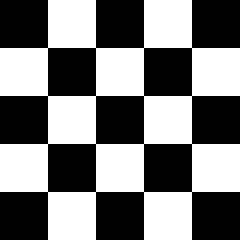This function can be expressed as a Fourier series,

$$f(\vec{r})=\sum \limits_{\vec{G}} f_{\vec{G}}\exp \left(i\vec{G}\cdot\vec{r}\right).$$

We choose the origin to be in the middle of one of the black squares. The Wigner-Seitz cell contains one black square so we integrate over the square to determine the Fourier coefficients,

$$f_{\vec{G}} = \frac{C}{a^2}\int\limits_{-\sqrt{2}a/4}^{\sqrt{2}a/4}\int\limits_{-\sqrt{2}a/4}^{\sqrt{2}a/4}\exp (-i\vec{G}\cdot \vec{r})dxdy.$$

By rewritting the exponential factor,

$$f_{\vec{G}} = \frac{C}{a^2}\int\limits_{-\sqrt{2}a/4}^{\sqrt{2}a/4}\int\limits_{-\sqrt{2}a/4}^{\sqrt{2}a/4}\exp (-iG_xx)\exp (-iG_yy)dxdy,$$

the integrals can easily be performed,

$$f_{\vec{G}} = \frac{C}{a^2}\frac{\left(\exp (-iG_x\sqrt{2}a/4)-\exp (iG_x\sqrt{2}a/4)\right)\left(\exp (-iG_y\sqrt{2}a/4)-\exp (iG_y\sqrt{2}a/4)\right)}{-G_xG_y}.$$

Using Euler's formula,

$$f_{\vec{G}} = \frac{C}{a^2}\frac{\left(\cos (-G_x\sqrt{2}a/4)+i\sin (-G_x\sqrt{2}a/4)-\cos (G_x\sqrt{2}a/4)-i\sin (G_x\sqrt{2}a/4)\right)\left(\cos (-G_y\sqrt{2}a/4)+i\sin (-G_y\sqrt{2}a/4)-\cos (G_y\sqrt{2}a/4)-i\sin (G_y\sqrt{2}a/4)\right)}{-G_xG_y}.$$

The cosine terms cancel out and the final result is,

$$f_{\vec{G}} = \frac{4C}{a^2}\frac{\sin (G_x\sqrt{2}a/4)\sin (G_y\sqrt{2}a/4)}{G_xG_y}.$$

Example 2: circles on a 2-d Bravais lattice

Consider a periodic function defined by non-overlapping circles arranged on a 2-D Bravais lattice.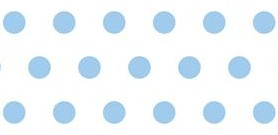A function $f$ is defined such that it has a constant value $C$ inside the circles and is zero outside the circles. As long as the circles do not overlap, a primitive unit cell can be defined so that the circle at the origin lies entirely within the primitive unit cell. Since the function $f$ is zero outside circle, we just need to integrate over the circle. The Fourier coefficients $f_{\vec{G}}$ are given by,

$$f_{\vec{G}} = \frac{C}{\pi R^2}\int\limits_{\text{circle}}\exp (-i\vec{G}\cdot \vec{r})d^2r = \frac{1}{\pi R^2}\int\limits_{0}^R\int\limits_{-\pi}^{\pi}\left(\cos (|G|r\cos\theta)- i\sin(|G|r\cos\theta)\right)rd\theta dr$$

Here $R$ is the radius of the circle. Performing the integral over $\theta$.

$$f_{\vec{G}} = \frac{2C}{R^2}\int\limits_{0}^RrJ_0(|G|r)dr$$

Here $J_0$ is the zeroth order Bessel function. Integrating over $r$ yields,

$$f_{\vec{G}} = \frac{2 C J_1(|G|R)}{R|G|}$$

Here $J_1$ is the first order Bessel function. As long as the circles do not overlap, the Fourier series for circles repeated on any 2-D Bravais lattice is,

$$f(\vec{r})=\frac{2 C }{R}\sum \limits_{\vec{G}} \frac{J_1(|G|R)}{|G|}\cos \left(i\vec{G}\cdot\vec{r}\right).$$

The sine terms cancel out since $f(\vec{r})$ is an even function.

Example 3: parallelograms repeated on a 2-D Bravais lattice

Consider a periodic function defined by non-overapping parallelograms arranged on a 2-D Bravais lattice.A function f is defined such that it has a constant value C inside the parallelograms and is zero outside the parallelograms. As long as the parallelograms do not overlap, a primitive unit cell can be defined so that the parallelogram at the origin lies entirely within the primitive unit cell. Since the function f is zero outside parallelogram, we just need to integrate over the parallelogram. The Fourier coefficients fG are given by,Here A is the area of the primitive unit cell. b and h are shown in the drawing. Performing the integral over y,Integrating over x yields,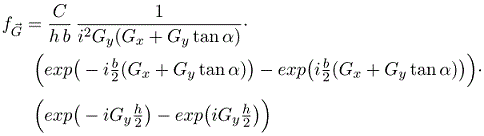As long as the parallelograms do not overlap, the Fourier series for parallelograms repeated on any 2-D Bravais lattice is,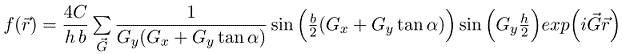This includes the results for squares and rectangles as special cases (tan(0) = 0). e.g. rectangle: